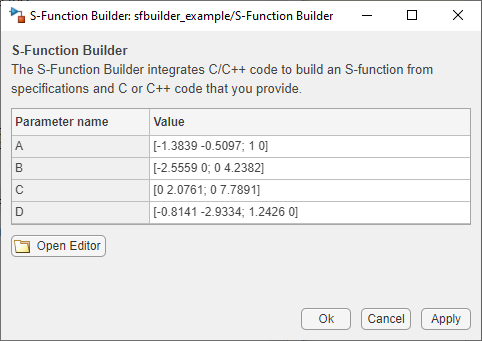## Model a State-Space System Using S-Function Builder

In this example, you will use the basic methods in the S-Function Builder to model a two-input, two-output discrete state-space system with two states. The state-space matrices are parameters to the S-function, and the S-function input and output are vectors.

You can investigate this example from `sfbuilder_example`. If you would like to study a manually written version of the created S-function, see `dsfunc.c`. Note that to create a S-function from an example S-Function Builder model, you need to build the model first.

### Configure the S-Function Builder Settings

Specify the number of discrete states and their initial conditions, the sample mode, and the sample time of the S-function. This example contains two discrete states, each initialized to `1`, and a discrete sample mode with a sample time of `1`. Ensure that the Direct feedthrough is selected, because the current values of the S-function inputs are used to compute its outputs.### Define Ports and Parameters

Use the Ports and Parameters table on the bottom of the editor to specify the ports and parameters of the S-function. For this example, we have one input, one output port, and four parameters.

To set or change the values of the block parameters, you can:

• Double-click the S-Function Builder block on the model.

• Use the Block Parameters from the context menu.

Alternatively, you can store the state-space matrices in variables in the MATLAB® workspace and enter the variable names into the Value field for each parameter. Enter the values in the image for state-space parameters on the Value field of the Block Parameters table.### Define the Output Method

In this example, The `Outputs_wrapper` method calculates the S-function output as a function of the input and state vectors and the state-space matrices. In the outputs code, reference S-function parameters using the parameter names defined on the Ports and Parameters table. Index into 2-D matrices using a scalar index, again keeping in mind that S-functions use zero-based indexing. For example, to access the element `C(2,1)` in the S-function parameter `C`, use `C`in the S-function code.

 ```void dsfunc_builder_Outputs_wrapper(const real_T *u, real_T *y, const real_T *xD, const real_T *xC, const real_T *A, const int_T p_width0, const real_T *B, const int_T p_width1, const real_T *C, const int_T p_width2, const real_T *D, const int_T p_width3) { /* Output_BEGIN */ y=C*xD+C*xD+D*u+D*u; y=C*xD+C*xD+D*u+D*u; /* Output_END */ }```

### Define the Update Method

The `Update_wrapper` method updates the discrete states. As with the outputs code, use the S-function parameter names and index into 2-D matrices using a scalar index, keeping in mind that S-functions use zero-based indexing. For example, to access the element `A(2,1)` in the S-function parameter `A`, use `A`in the S-function code. The variable `xD` stores the final values of the discrete states. Enter the following code in the `Update_wrapper` function.

 ```void dsfunc_builder_Update_wrapper(const real_T *u, real_T *y, real_T *xD, const real_T *A, const int_T p_width0, const real_T *B, const int_T p_width1, const real_T *C, const int_T p_width2, const real_T *D, const int_T p_width3) { /* Update_BEGIN */ real_T tempX = {0.0, 0.0}; tempX=A*xD+A*xD+B*u+B*u; tempX=A*xD+A*xD+B*u+B*u; xD = tempX; xD = tempX; /* Update_END */ }```

### Build the State-Space System

Click the arrow under `Build` and select the following options:

• Show compile steps

• Create a debuggable MEX-file

• Generate wrapper TLC

To build your S-function, click Build on the toolstrip to create an executable file for this S-function. You can now run the model and compare the output to the original discrete state-space S-function contained in `sfcndemo_dsfunc`.